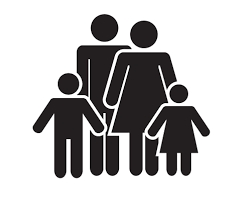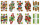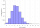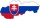# Families 2

Seven hundred twenty-nine families are having six children each. The probability of a girl is 1/3, and the probability of a boy is 2/3. Find the number of families having two girls and four boys.

n =  240

### Step-by-step explanation:Did you find an error or inaccuracy? Feel free to write us. Thank you!Math student
The correction notation is p = 6 combination 2 and not 2 combination 6Matematik
Thanks, we just exange 6 and 2Tips to related online calculators
Looking for a statistical calculator?
Need help to calculate sum, simplify or multiply fractions? Try our fraction calculator.
Would you like to compute count of combinations?

## Related math problems and questions:

• Boys and girlsThere are 11 boys and 18 girls in the classroom. Three pupils will answer. What is the probability that two boys will be among them?
• Family94 boys are born per 100 girls. Determine the probability that there are two boys in a randomly selected family with three children.
• Combinations 66 purses 9 flaps 12 straps Every combination must include 1 purse, 1 flap, and 1 strap. How many are possible combinations?
• Boys and girlsThere are eight boys and nine girls in the class. There were six children on the trip from this class. What is the probability that left a) only boys b) just two boys
• ClassroomOf the 26 pupils in the classroom, 12 boys and 14 girls, four representatives are picked to the odds of being: a) all the girls b) three girls and one boy c) there will be at least two boys
• FamilyWhat is the probability that a family with 7 childrens have: exactly 6 dívek? 2 dívky and 5 boys? Consider the birth probability of a girl is 48.87% and boy 51.13%.
• Created trioWhat is the probability that in the created trio, which consists of 19 boys and 12 girls, they will be: a) the boys themselves b) the girls themselves c) 2 boys and 1 girl?
• DivisionDivision has 18 members: 10 girls and 6 boys, 2 leaders. How many different patrols can be created, if one patrol is 2 boys, 3 girls and 1 leader?
• Playing cardsFrom 32 playing cards containing 8 red cards, we choose 4 cards. What is the probability that just 2 will be red?
• CardsFrom a set of 32 cards, we randomly pull out three cards. What is the probability that it will be seven kings and ace?
• DiceWe throw 10 times a playing dice. What is the probability that the six will fall exactly 4 times?
• DiceWe throw five times the dice. What is the probability that six fits exactly twice?
• Binomial probabilityWhat is the binominal probability that at least 4 of the 6 trials (n=6) are succeeded where φ = 0.50?
• STRESSED wordEach letter in STRESSED is printed on identical cards, one letter per card, and assembled in random order. Calculate the probability that the cards spell DESSERTS when assembled.
• A marriedA married couple planned to have 3 children. i. List the possible combinations of the sexes of 3 children. Use B for boy and G for girl. ii. Calculate the probability that all three children would be of the same gender
• School parliamentThere are 18 boys and 14 girls in the class. In how many ways can 3 representatives be elected to the school parliament, if these are to be: a) the boys themselves b) one boy and two girls
• Three workplacesHow many ways can we divide nine workers into three workplaces if they need four workers in the first workplace, 3 in the second workplace, and 2 in the third?